Algebra 2 1-1 Guided Practice: Patterns and Expressions
starstarstarstarstarstarstarstarstarstar
by Matthew Richardson
| 13 Questions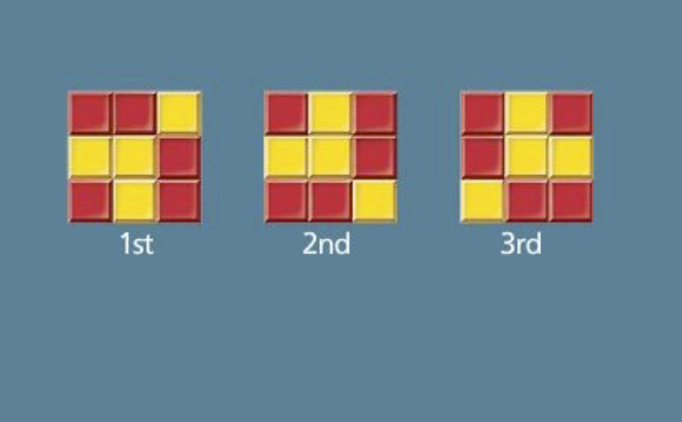1
1
10
Solve It! You are playing a video game. You reach a locked gate. The lock is square with 9 sections. You can make a key by placing a red or yellow block in each section. Near the gate is a carving of a pattern of squares.

The key to the gate is the eighth image in the pattern. Sketch the key to the gate on the canvas.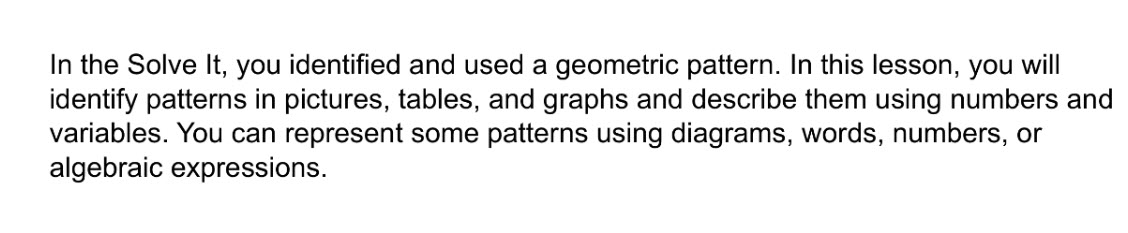2
3
2
10
Problem 1 Got It? Look at the figures from left to right. What is the pattern?
3
10
Problem 1 Got It? Look at the figures from left to right. Sketch the next figure in the pattern on the canvas.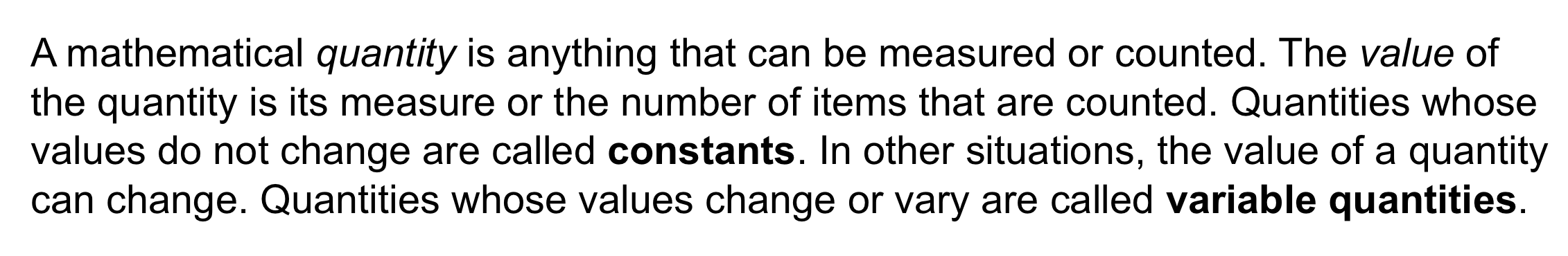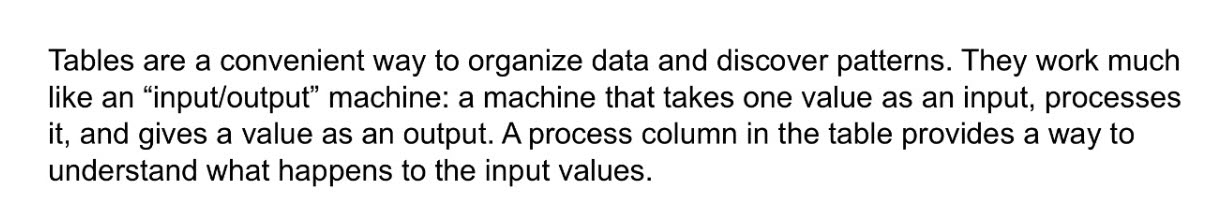4
5
6
7
8
4
10
Take Note: Define constants as they apply to this lesson.
5
10
Take Note: Define variable quantities (aka variables).
6
10
Take Note: Provide an example of a numerical expression.
7
10
Take Note: Provide an example of an algebraic expression.
8
10
Take Note: What is a process column and how is it helpful?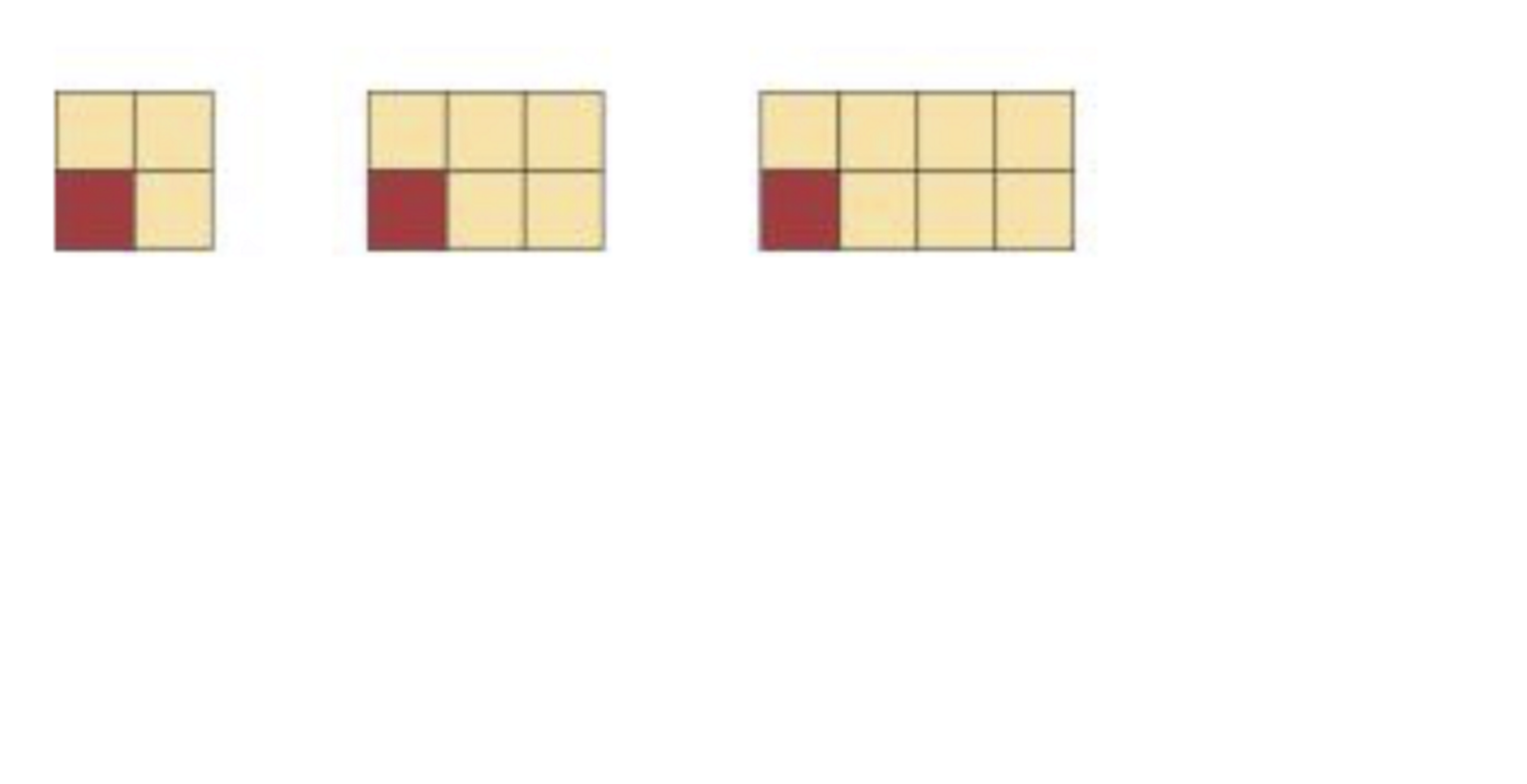9
9
10
Problem 2 Got It? How many tiles are in the 25th figure in this pattern? Use a table of values with a process column.

Respond with only a number and no spaces.a
10
11
12
Problem 3 Got It? The graph shows the cost of platys at the aquarium shop. Use a table to answer the questions.
10
10
Problem 3 Got It? How much do six platy's cost? Enter only a whole dollar amount with a dollar sign. Use this format: \$21
11
10
Problem 3 Got It? How much do ten platy's cost? Enter only a whole dollar amount with a dollar sign. Use this format: \$21
12
10
Problem 3 Got It? Reasoning: Why is the graph in Problem 3, shown below, a line while the graph shown here is a set of points?13
13
10
Take Note: Summarize the mathematical content of this lesson. What topics, ideas, and vocabulary were introduced?